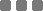## Thursday, October 15, 2015

### Python: Convert List into String

The method keyword is similar like in `JavaScript`. Both are using the `join` keyword. But in `Python`, the syntax is reversed (or not, depends on your perspective).

In `JavaScript`

For example:

In the example above, the `array` input is converted into strings output with one horizontal white space as the "punctuation" (separator).

In `Python`

For example:

In the example above, the `list` input is converted into strings output also with one horizontal white space as the "punctuation" (separator).

Unlike in `JavaScript`, which we can convert an `array` with mixed types of elements (nonuniform) to string, the `join()` method in `Python` only works for `list` which consists of only string (such as the example above).

To join those mixed element types, first we need to convert each element into string using `str()` method. Then we can `join()` them. Let's see an example below.

Converting `list` with mixed types of elements to `string`

Let's use and expand the `JavaScript` example input:

So the iteration shorthand for converting each `list` element into string is this marked part:

`" ".join(str(elm) for elm in myOtherList)`

The method above won't change the original `list`.

For no reason, we can also separate the iteration and conversion, then make a function for it. Let's name the function as convertToString. Here it goes:

This method also won't change the original `list`. As you can see, there's that "buffer" variable.
Very "exploration" *clap clap clap

Copy List

This is a neat reference of various methods and shorthand to copy a list in Python (slice an array - in JavaScript) on StackOverflowPython: Convert List into String
https://monkeyraptor.johanpaul.net/2015/10/python-convert-list-into-string.html?m=0